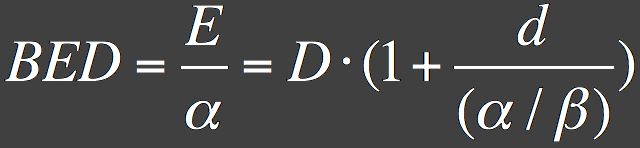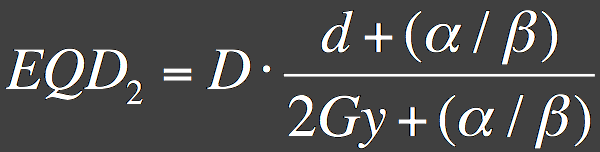### EquationsBED = biologically effective dose
EQD2 = dose in 2 Gy fractions biologically equivalent to dose D given in fractions of size d, in units of Gray

E is biological effect, D is total dose (D = nd), d is dose per fraction, n is the number of fractions, and alpha/beta is a property of the irradiated tissue.